By going through these CBSE Class 12 Maths Notes Chapter 6 Application of Derivatives, students can recall all the concepts quickly.

## Application of Derivatives Notes Class 12 Maths Chapter 6

Rate of change of Quantities: Let y =f(x) be a function. If the change in one quantity y varies with another quantity x, then $$\frac{d y}{d x}$$ = f ‘ (x) denotes the rate of change of y with respect to x. At x = x0$$\left.\frac{d y}{d x}\right]_{x=x_{0}}$$, or f'(x) represents the rate of change of y w.r.t. x at x = x0.

Let I will be the open interval contained in the domain of real-valued function f.

Increasing Function: f is said to be increasing function on I, if x1 < x2 on I, then f(x1) ≤ f(x2) for all x1, x2 ∈ I.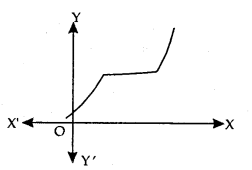Strictly Increasing Function: f is said to be strictly increasing on I if x1 < x2 in I ⇒ f(x1) < f(x2) for all x1, x2 ∈ I.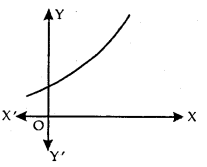Decreasing Function: f is said to be decreasing function on I, if x1 < x2 in I, then f(x1) ≥ f(x2) for all x1, x2 ∈ I.Strictly Decreasing Function: f is said to be strictly decreasing function on I, if x1 < x2 in I ⇒ f(x1) > f(x2) for all x1, x2 ∈ I.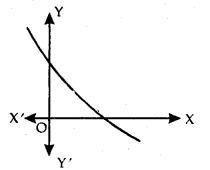Increasing and Decreasing Functions at x0:
Let x0 be a point in the domain of definition of a real valued function f and there exists an open interval I = (x0 – h, x0 + h) containing x0 such that

1. f is increasing at x0, if x1 < x2 in I ⇒ f(x1) ≤ f(x2).
2. f is strictly increasing at x0, if x1 < x2 in I ⇒ f(x1) < f(x2).
3. f is decreasing at x0, if x1 < x2 in I ⇒ f(x1) ≥ f(x2).
4. f is strictly decreasing at x0, if x1 < x2 ⇒ f(x1) >f(x2).

Test: Increasing/decreasing/constant Functions:
Let f be continuous on [a, b] and differentiable in an open interval (a, b), then

1. f is increasing on [a, b], if f ‘(x) > 0 for each x ∈ (a, b).
2. f is decreasing on [a, b], if f ‘(x) < 0 for each x ∈ (a, b).
3. f is constant on [a, b], if f ‘(x) = 0 for each x ∈ (a, b).

→ Tangent to a curve: Let y = f(x) be the equation of a curve. The equation of the tangent at (x0, y0) is y – y0 = m(x – x0),
where m = slope of the tangent
= $$\left.\frac{d y}{d x}\right]_{\left(x_{0}, y_{0}\right)}$$ = f ‘(x0).

→ Normal to the curve: Let y = f(x) be the equation of a curve.
Equation of the normal is
y – y0 = – $$\frac{1}{m}$$(x – x0)
or
x – x0 + m(y – y0) = 0,
where m = slope of the tangent
= $$\left.\frac{d y}{d x}\right]_{\left(x_{0}, y_{0}\right)}$$ = f ‘(x0)

It may be noted that, slope of normal
= – $$\frac{1}{m}=\frac{-1}{f^{\prime}\left(x_{0}\right)}$$.

→ Particular case of tangent: Let m = tan θ. If θ = 0, then m = 0.
Equation of tangent is y – y0 = 0, i.e., y = y0.
If θ = $$\frac{π}{2}$$, m is not defined.
∴ (x – x0) = $$\frac{1}{m}$$ (y – y0).
when θ = $$\frac{π}{2}$$, then cot $$\frac{π}{2}$$ = 0.

∴ Equation of tangent is x – x0 = 0 or x = x0.

→ Approximation: Let f: D → R, D⊂R such that y = f(x) and Δy is the increment in y corresponding to increment Δx in x, where Δy = f(x + Δx) – f(x).
Now,

1. the differential of x denoted by dx is defined by dx = Δx
2. The differential of y, denoted by dy, is defined by
dy = f ‘(x)dx
or
dy = ($$\frac{dy}{dx}$$)Δx

Maximum value, Minimum Value, Extreme Value:
Let f be a function defined in the interval I. Then,
1. Maximum Value:
If there exists a point x = c in I such that f(c) > f(x), for all x ∈ I, then/is maximum in I. Point c is known as a point of the maximum value in I.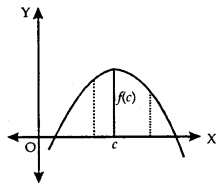2. Minimum Value:
If there exists a point x = c in I such that f(c) ≤ f(x), for all x ∈ I, then/is minimum in I. Point c is called as a point of the minimum value in I.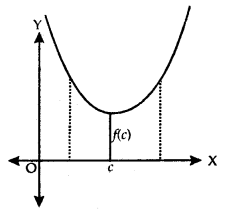3. Extreme Value:
If there exists a point x = c in I such that f(c) is either a maximum value or a minimum value in I, then f is having an extreme value in I. Point c is said to be an extreme point.Local Maxima and Minima:
Let f be a real-valued function and x = c be an interior point in the domain of f, then
(a) Local Maxima: c is a point of local maxima, if there is an h > 0, such that f(c) ≥ f(x) for all x ∈ (c – h, c + h).
The value f(c) is called the local maximum value of f.

(b) Local Minimum: c is a point of local minimum, if there is an h > 0, such that f(c) ≤ f(x) for all x ∈ (c – h, c + h).
The value of f(c) is known as the local minimum value of f.

Geometrically if x = c is a point of local maxima of f, then f is increasing (f ‘(x) > 0) in the interval (c – h, c) and decreasing (f ‘(x) < 0) in the interval (c, c + h)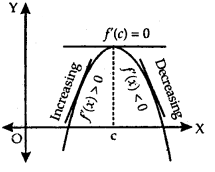This implies f ‘(c) = 0.

Test of Maxima and Minima:
1. Let f be a function on an open interval I and c ∈ I will be any point. If f has local maxima or local minima at x = c, then either f ‘(c) = 0 or f is not differentiable at c.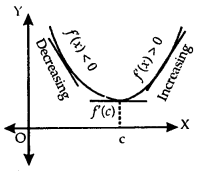Let f be continuous at a critical point c in it.

2. If f ‘(x) changes sign from positive to negative as x increases through c, i.e.,

1. (i) f ‘(x) > 0 at every point in (c – h, c) and
2. (ii) f ‘(x) < 0 at every point in (c, c + h), where h is sufficiently small, then there is a point of local maxima.

3. If f ‘(x) changes sign from negative to positive as x increases through c, i.e.,

1. f(x) < 0 at every point in (c – h, c) and
2. f ‘(x) > 0 at every point in (c, c + h), where It is sufficiently small, then c is a point of local minima.

4. If f ‘(x) does not change significantly as x increase through c, then c is neither a point of local maxima nor a point of local minima. Such a point is called point of inflection.

Second Derivative Test of Maxima and Minima:
Let f be a function defined on an interval I and c ∈ I and f be differentiable at c. Then,

1. Maxima: x = c is a local maxima, if f'(c) = 0 and f “(c) < 0.
2. Minima: x = c is a local minima, iff'(c) = 0 and f “(c) > 0. Here, f(c) is the local minimum value off.
3. Point of Inflection: If f ‘(c) = 0 and f “(c) = 0, then test fails. Then, we apply the first derivative test as x increases through c.

Maximum and Minimum Values in a Closed Interval:
Consider the function f(x) = x+ 3, x ∈ [0,1]. Here f ‘(c) ≠ 0. It has neither maxima nor minima. But f(0) = 3. This is the absolute minimum or global minimum or least value.

Further, f(1) = 4. This is the absolute maximum or global maximum or greatest value.
Further, consider f be any other continuous function having local maxima and minima.

Absolute Maxima and Minima:
Let f be a continuous YA function on an interval I = [a, b].
Then, f has the absolute maximum value and/attains it at least once in I. Similarly,f has the absolute minimum value and attains at least once in I.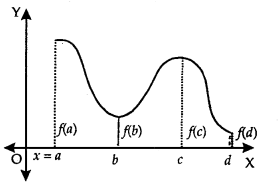At x = b, there is a minimum.
At x = c, there is a maxima.
At x = a, f(a) is the greatest value or absolute maximum value.
– At x = d,f(d) is the least value or absolute minimum value.

To find absolute maximum value or absolute minimum value:

1. Find all the critical points viz. where f ‘ (x) = 0 or f is not differentiable.
2. Consider the endpoints also.
3. Calculate the values of the function at all the points found in steps (1) and (2).
4. Identify the maximum and minimum values out of the values calculated in step 3. These are absolute maximum and absolute minimum values.

1. DERIVATIVEASARATEMEASURE
f'(x) is the rate measure of fix) w.r.t. x.

2. INCREASING AND DECREASING FUNCTIONS

(i) A function f(x) is said to be increasing function of x
if x1 ≤ x2 ⇒ f(x1) ≤ f(x2)
or x1 ≥ x2 ⇒ f(x1) ≥ f(x2)

(ii) A function f(x) is said to be a strictly increasing function of x
if x1 < x2 ⇒ f(x1) < f(x2)
or x1 > x2 ⇒ f(x1) > f(x2)

(iii) A function f(x) is said to be a decreasing function of x,
if x1 ≤ x2 ⇒ f(x1) ≥ f(x2)
or x1 ≥ x2 ⇒ f(x1) ≤ f(x2)

(iv) A function f(x) is said to be a strictly decreasing function of x,
if x1 < x2 ⇒ f(x1) > f(x2)
or x1 > x2 ⇒ f(x1) < f(x2)

(v) Monotone Function. A function is said to be monotone if it is either increasing or decreasing.

3. TANGENTSANDNORMALS

(i) lf y = f(x), then the slope of the tangent at P (x = c) is $$\left.\frac{d y}{d x}\right]_{x=c}$$

(ii) Equation of the normal at P(x’, y’) to the curve y = f(x) is y – y’ =$$\left(\frac{d y}{d x}\right)_{\mathrm{P}}$$ (x – x’)

(iii) Equation of the normal at P(x’, y’) to the curve y = f(x) is y – y’= $$-\frac{1}{\left(\frac{d y}{d x}\right)_{\mathrm{P}}}$$ (x – x’)

4. MAXIMA AND MINIMA

(i) Method to find absolute max. and min., values in a given interval.
(a) Find all points when $$\frac{d y}{d x}$$ =0.
(b) Take end points of the interval.
(c) At all these points, calculate values of y.
(d) Take the maximum and minimum values out of these values.

(ii) If f(a) is an extreme value of f(x), then f'(a) = 0.

(iii) Local Maximum and Minimum values.
(a) First Derivative Test GUIDE-LINES:
Let y=f(x).
Step (i) Put $$\frac{d y}{d x}$$ = 0. Solve it for getting x = a, b, c,… dx

Step (ii) Select x = a.
Study the sign of $$\frac{d y}{d x}$$ when (I) x < a slightly dx (II) x > a slightly.
(a) If the former is + ve and latter is -ve, then f(x) is max. at x = a.
(b) If the former is – ve and latter is +ve, then f(x) is min. at x = a.

Step (iii) Putting those values of x for which f(x) is max. or min. and get the corresponding max. or min. values of f(x).

(b) Second Derivative Test GUIDE-LINES:
Step (i) Put y = f(x) and find $$\frac{d y}{d x}$$ i.e., f(x).

Step (ii) Put $$\frac{d y}{d x}$$ = 0 i.e. f'(x) = 0 and solve it for x giving 😡 = a, b, c,…

Step (iii) Select x = a. Find $$\frac{d^{2} y}{d x^{2}}$$ i.e. f”(x) at x = a.

(I) If $$\left.\frac{d^{2} y}{d x^{2}}\right]_{x=a}$$ i. e. f”(a) is -ve, then x = a gives the max. value.
(II) If $$\left.\frac{d^{2} y}{d x^{2}}\right]_{x=a}$$i.e. f”(a) is +ve, then x = a gives the min. value.
Similarly for x = b, c,…